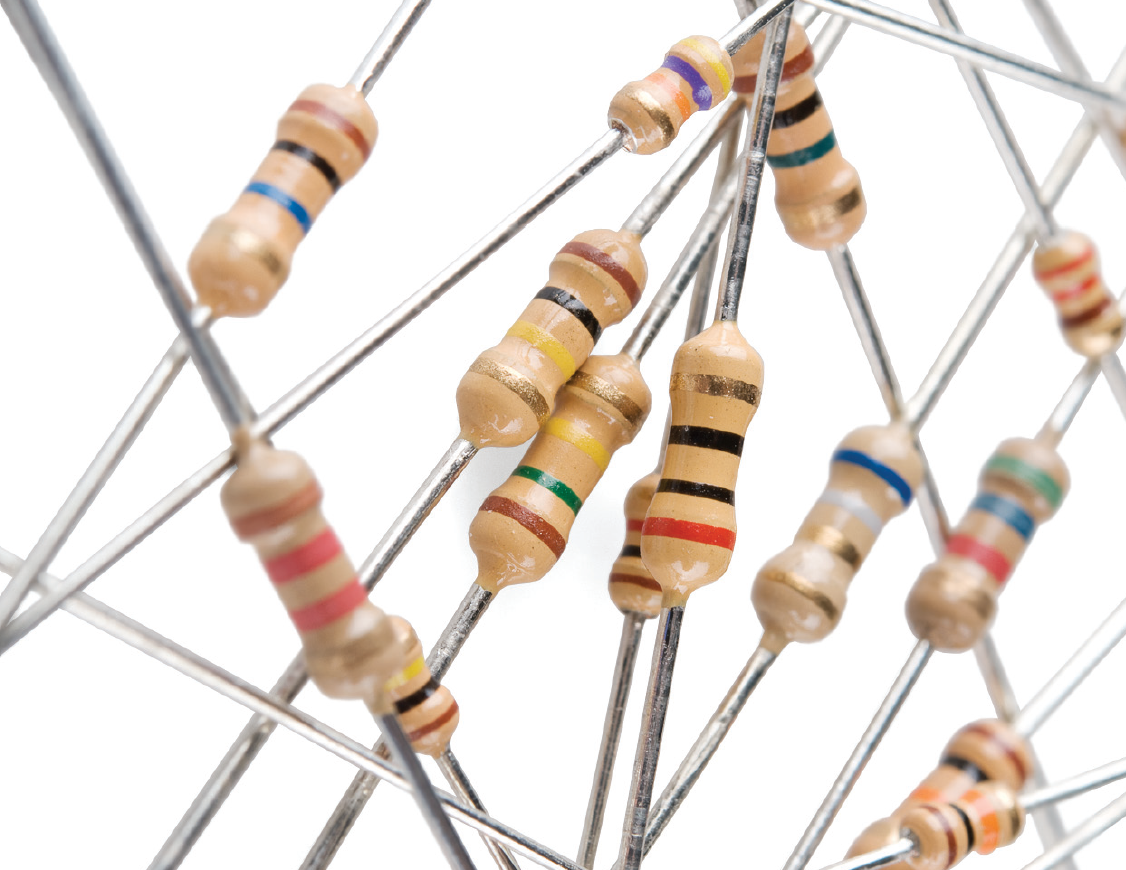# Understanding Resistors

Resistors are in nearly every circuit. They help to regulate the flow of electricity to our components and can protect sensitive components too.

They do what their name suggests: they resist the flow of electricity. Each resistor does this to a specific amount, known as their resistance. This is measured in Ohms (denoted as Ω) and is based on Ohm’s law (see What is Electricity).

# Prototyping with Resistors

While in normal circuits, you’ll want to use resistors more precisely; when prototyping we really only need two resistors: 1KΩ and a 10KΩ. The 1KΩ resistor is normally placed before components like LEDs to protect them while the 10KΩ resistor is used to impede the flow of electricity but create a complete when working with switches and other input devices. More on that later…Resistors of various resistances - color bands denote how much resistance they give

# Knowing which resistor is which

All resistors are color coded. The have four bands which you can use to tell you how much resistance they have

The first two bands tells you the most important digits of the resistors value. The third band is a multiplier or a weight, which multiplies the previous two digits by a power of ten. The final band is the tolerance of the resistor or the degree of accuracy in its resistance. A 1% tolerance means it could be -1% or +1% of the amount specified.

Confused? Don’t be. You don’t really need to know how to look up resistance for two reasons:

1. You can use a calculator like this one to find out the resistance based on the band colors: http://www.digikey.com/en/resources/conversion-calculators/conversion-calculator-resistor-color-code-4-band

2. Or, I can just tell you the color codes of the two resistors you’ll use most often:

1. 1KΩ: **BROWN, BLACK, **RED, [GOLD]

2. 10KΩ: BROWN, BLACK, ORANGE, [GOLD]

# Using a resistor

Every resistor has two terminals (or wires). It doesn’t matter which direction, order, orientation, etc you put these wires in, it will work either way!